# Java Program to Calculate Batting Average

In this tutorial you will learn about the Java Program to Calculate Batting Average and its application with practical example.

In this tutorial, we will learn to create a Java Program to Calculate Batting Average using Java programming.

## Prerequisites

Before starting with this tutorial we assume that you are best aware of the following Java programming topics:

• Java Operators.
• Basic Input and Output function in Java.
• Class and Object in Java.
• Basic Java programming. Basic Java programming.
• If-else statements in Java.
• For loop in Java.

Batting Average.

By taking three values ” runsmatches, and number of not-out” represent number of runs scored and innings played by the batsman and number of times remained Not Out. respectively.For calculating Batting Average

“Average= Run score/number of dismissals.”

where : number of dismissals = number of innings -number of innings remained not out.

## Java Program to Calculate Batting Average

In this program we will to create a program to calculate Bating Average using java program. We would first declared and initialized the required variables. Next, we would prompt user to input the values.Later we find Batting average.

## Output

Batting Average.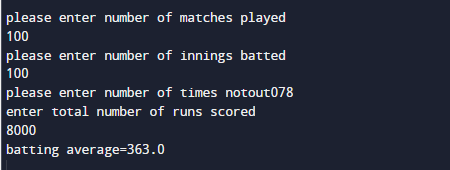In the above program, we have first declared and initialized a set variables required in the program.

• match = it will hold entered match
• run   =  it will hold entered runs
• ings  = it will hold entered innings.
• notout= it will hold total number of not-out.

And in the next statement user will be prompted to enter  different values  and  which will be assigned to variables “match,run , notout , ings” respectevely.

Matches: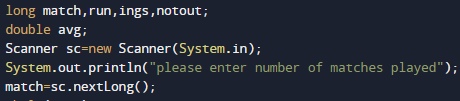Innings: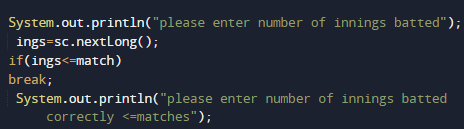Not-out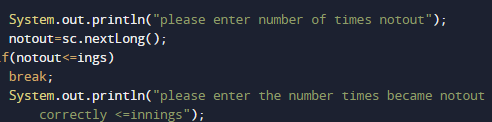Runs: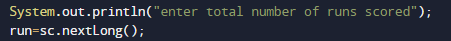After taking all the values,Now we will calculate Bating average.
First we calculate the number of dismissals,that’s equal to matches – notout.
Batting Average, equal to runs/ (matches – notout).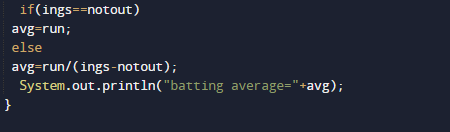At last we will Print the calculated Bating average.In this tutorial we have learn about the Java Program to Calculate Batting Average and its application with practical example. I hope you will like this tutorial.